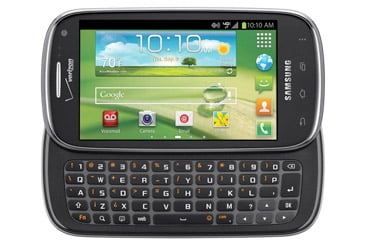-=+=- -=+=- -=+=- -=+=- -=+=- -=+=- -=+=- -=+=- -=+=- -=+=- -=+=- -=+=- -=+=- -=+=- -=+=- -=+=- -=+=- -=+=- -=+=- -=+=- -=+=- -=+=- -=+=- -=+=- -=+=- -=+=- -=+=- -=+=- -=+=- -=+=- (c) WidthPadding Industries 1987 0|417|0 -=+=- -=+=- -=+=- -=+=- -=+=- -=+=- -=+=- -=+=- -=+=- -=+=- -=+=- -=+=- -=+=- -=+=- -=+=- -=+=- -=+=- -=+=- -=+=- -=+=- -=+=- -=+=- -=+=- -=+=- -=+=- -=+=- -=+=- -=+=- -=+=- -=+=-
Socoder -> Hardware and Technology -> GPD Win 3

Posted : Sunday, 08 November 2020, 03:31
Jayenkai### GPD Win 3On the plus side, it's roughly the size of a Switch Lite, and .. boy, does that look neat and compact.

My main concern is..Aaargh! Squared Keyboard!!!!
Z does NOT go below Q...

-=-=-Posted : Sunday, 08 November 2020, 06:51
rychanYou do love those interesting small form factors, I totally get itreminds me of a qwerty keyboard phone i had about 10 years ago which had a slide up screen also, bloody loved that one!

-=-=-
Web / Game Dev, occasionally finishes off coding games also!

Refresh Games - Game Dev BlogPosted : Sunday, 08 November 2020, 07:02
rockfordreminds me of a qwerty keyboard phone i had about 10 years ago which had a slide up screen also, bloody loved that one!I had (and still have) a Palm Pre 2 (and later a Pre and Pixi) for developing. They were all really great phones, for the time. I really do miss their physical keyboards.
Posted : Sunday, 08 November 2020, 13:35
rychanYeah, something like this image:-=-=-
Web / Game Dev, occasionally finishes off coding games also!

Refresh Games - Game Dev BlogPosted : Wednesday, 06 January 2021, 03:30
JayenkaiMmmm...

Would love to hear (and/or not hear) the sound of the fan, irl.. that's the one thing that disappointed me with the original GPD Win.

-=-=-